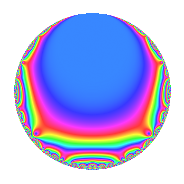Properties

 Label 9450.2.a.erLevel 9450 Weight 2 Character orbit 9450.a Self dual Yes Analytic conductor 75.459 Analytic rank 0 Dimension 2 CM No Inner twists 1

Related objects

Newspace parameters

 Level: $$N$$ = $$9450 = 2 \cdot 3^{3} \cdot 5^{2} \cdot 7$$ Weight: $$k$$ = $$2$$ Character orbit: $$[\chi]$$ = 9450.a (trivial)

Newform invariants

 Self dual: Yes Analytic conductor: $$75.4586299101$$ Analytic rank: $$0$$ Dimension: $$2$$ Coefficient field: $$\Q(\sqrt{3})$$ Coefficient ring: $$\Z[a_1, \ldots, a_{11}]$$ Coefficient ring index: $$1$$ Fricke sign: $$-1$$ Sato-Tate group: $\mathrm{SU}(2)$

$q$-expansion

Coefficients of the $$q$$-expansion are expressed in terms of $$\beta = \sqrt{3}$$. We also show the integral $$q$$-expansion of the trace form.

 $$f(q)$$ $$=$$ $$q$$ $$+ q^{2}$$ $$+ q^{4}$$ $$- q^{7}$$ $$+ q^{8}$$ $$+O(q^{10})$$ $$q$$ $$+ q^{2}$$ $$+ q^{4}$$ $$- q^{7}$$ $$+ q^{8}$$ $$+ \beta q^{11}$$ $$+ ( 2 + 2 \beta ) q^{13}$$ $$- q^{14}$$ $$+ q^{16}$$ $$+ 4 q^{17}$$ $$+ ( 4 + \beta ) q^{19}$$ $$+ \beta q^{22}$$ $$+ ( -1 + 2 \beta ) q^{23}$$ $$+ ( 2 + 2 \beta ) q^{26}$$ $$- q^{28}$$ $$+ ( -2 + 2 \beta ) q^{29}$$ $$+ ( -2 + \beta ) q^{31}$$ $$+ q^{32}$$ $$+ 4 q^{34}$$ $$+ ( 2 - 3 \beta ) q^{37}$$ $$+ ( 4 + \beta ) q^{38}$$ $$+ ( 3 - 4 \beta ) q^{41}$$ $$+ ( -8 + 2 \beta ) q^{43}$$ $$+ \beta q^{44}$$ $$+ ( -1 + 2 \beta ) q^{46}$$ $$+ ( 8 + 2 \beta ) q^{47}$$ $$+ q^{49}$$ $$+ ( 2 + 2 \beta ) q^{52}$$ $$-8 \beta q^{53}$$ $$- q^{56}$$ $$+ ( -2 + 2 \beta ) q^{58}$$ $$+ ( -2 + 4 \beta ) q^{59}$$ $$-8 \beta q^{61}$$ $$+ ( -2 + \beta ) q^{62}$$ $$+ q^{64}$$ $$+ ( -2 - 6 \beta ) q^{67}$$ $$+ 4 q^{68}$$ $$+ ( 6 - \beta ) q^{71}$$ $$+ ( 10 + 4 \beta ) q^{73}$$ $$+ ( 2 - 3 \beta ) q^{74}$$ $$+ ( 4 + \beta ) q^{76}$$ $$- \beta q^{77}$$ $$+ ( 2 + 4 \beta ) q^{79}$$ $$+ ( 3 - 4 \beta ) q^{82}$$ $$+ ( 6 + 2 \beta ) q^{83}$$ $$+ ( -8 + 2 \beta ) q^{86}$$ $$+ \beta q^{88}$$ $$+ q^{89}$$ $$+ ( -2 - 2 \beta ) q^{91}$$ $$+ ( -1 + 2 \beta ) q^{92}$$ $$+ ( 8 + 2 \beta ) q^{94}$$ $$-4 \beta q^{97}$$ $$+ q^{98}$$ $$+O(q^{100})$$ $$\operatorname{Tr}(f)(q)$$ $$=$$ $$2q$$ $$\mathstrut +\mathstrut 2q^{2}$$ $$\mathstrut +\mathstrut 2q^{4}$$ $$\mathstrut -\mathstrut 2q^{7}$$ $$\mathstrut +\mathstrut 2q^{8}$$ $$\mathstrut +\mathstrut O(q^{10})$$ $$2q$$ $$\mathstrut +\mathstrut 2q^{2}$$ $$\mathstrut +\mathstrut 2q^{4}$$ $$\mathstrut -\mathstrut 2q^{7}$$ $$\mathstrut +\mathstrut 2q^{8}$$ $$\mathstrut +\mathstrut 4q^{13}$$ $$\mathstrut -\mathstrut 2q^{14}$$ $$\mathstrut +\mathstrut 2q^{16}$$ $$\mathstrut +\mathstrut 8q^{17}$$ $$\mathstrut +\mathstrut 8q^{19}$$ $$\mathstrut -\mathstrut 2q^{23}$$ $$\mathstrut +\mathstrut 4q^{26}$$ $$\mathstrut -\mathstrut 2q^{28}$$ $$\mathstrut -\mathstrut 4q^{29}$$ $$\mathstrut -\mathstrut 4q^{31}$$ $$\mathstrut +\mathstrut 2q^{32}$$ $$\mathstrut +\mathstrut 8q^{34}$$ $$\mathstrut +\mathstrut 4q^{37}$$ $$\mathstrut +\mathstrut 8q^{38}$$ $$\mathstrut +\mathstrut 6q^{41}$$ $$\mathstrut -\mathstrut 16q^{43}$$ $$\mathstrut -\mathstrut 2q^{46}$$ $$\mathstrut +\mathstrut 16q^{47}$$ $$\mathstrut +\mathstrut 2q^{49}$$ $$\mathstrut +\mathstrut 4q^{52}$$ $$\mathstrut -\mathstrut 2q^{56}$$ $$\mathstrut -\mathstrut 4q^{58}$$ $$\mathstrut -\mathstrut 4q^{59}$$ $$\mathstrut -\mathstrut 4q^{62}$$ $$\mathstrut +\mathstrut 2q^{64}$$ $$\mathstrut -\mathstrut 4q^{67}$$ $$\mathstrut +\mathstrut 8q^{68}$$ $$\mathstrut +\mathstrut 12q^{71}$$ $$\mathstrut +\mathstrut 20q^{73}$$ $$\mathstrut +\mathstrut 4q^{74}$$ $$\mathstrut +\mathstrut 8q^{76}$$ $$\mathstrut +\mathstrut 4q^{79}$$ $$\mathstrut +\mathstrut 6q^{82}$$ $$\mathstrut +\mathstrut 12q^{83}$$ $$\mathstrut -\mathstrut 16q^{86}$$ $$\mathstrut +\mathstrut 2q^{89}$$ $$\mathstrut -\mathstrut 4q^{91}$$ $$\mathstrut -\mathstrut 2q^{92}$$ $$\mathstrut +\mathstrut 16q^{94}$$ $$\mathstrut +\mathstrut 2q^{98}$$ $$\mathstrut +\mathstrut O(q^{100})$$

Embeddings

For each embedding $$\iota_m$$ of the coefficient field, the values $$\iota_m(a_n)$$ are shown below.

For more information on an embedded modular form you can click on its label.

Label $$\iota_m(\nu)$$ $$a_{2}$$ $$a_{3}$$ $$a_{4}$$ $$a_{5}$$ $$a_{6}$$ $$a_{7}$$ $$a_{8}$$ $$a_{9}$$ $$a_{10}$$
1.1
 −1.73205 1.73205
1.00000 0 1.00000 0 0 −1.00000 1.00000 0 0
1.2 1.00000 0 1.00000 0 0 −1.00000 1.00000 0 0
 $$n$$: e.g. 2-40 or 990-1000 Significant digits: Format: Complex embeddings Normalized embeddings Satake parameters Satake angles

Inner twists

This newform does not admit any (nontrivial) inner twists.

Atkin-Lehner signs

$$p$$ Sign
$$2$$ $$-1$$
$$3$$ $$-1$$
$$5$$ $$-1$$
$$7$$ $$1$$

Hecke kernels

This newform can be constructed as the intersection of the kernels of the following linear operators acting on $$S_{2}^{\mathrm{new}}(\Gamma_0(9450))$$:

 $$T_{11}^{2}$$ $$\mathstrut -\mathstrut 3$$ $$T_{13}^{2}$$ $$\mathstrut -\mathstrut 4 T_{13}$$ $$\mathstrut -\mathstrut 8$$ $$T_{17}$$ $$\mathstrut -\mathstrut 4$$ $$T_{19}^{2}$$ $$\mathstrut -\mathstrut 8 T_{19}$$ $$\mathstrut +\mathstrut 13$$